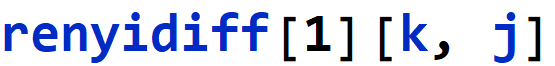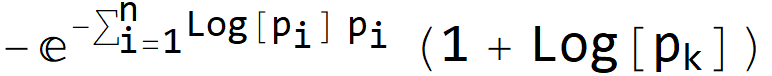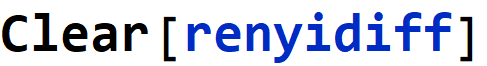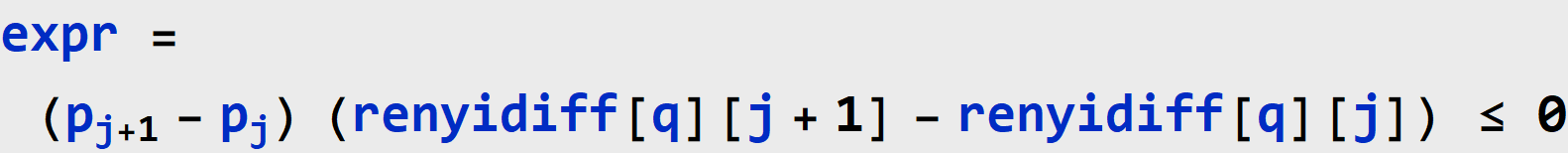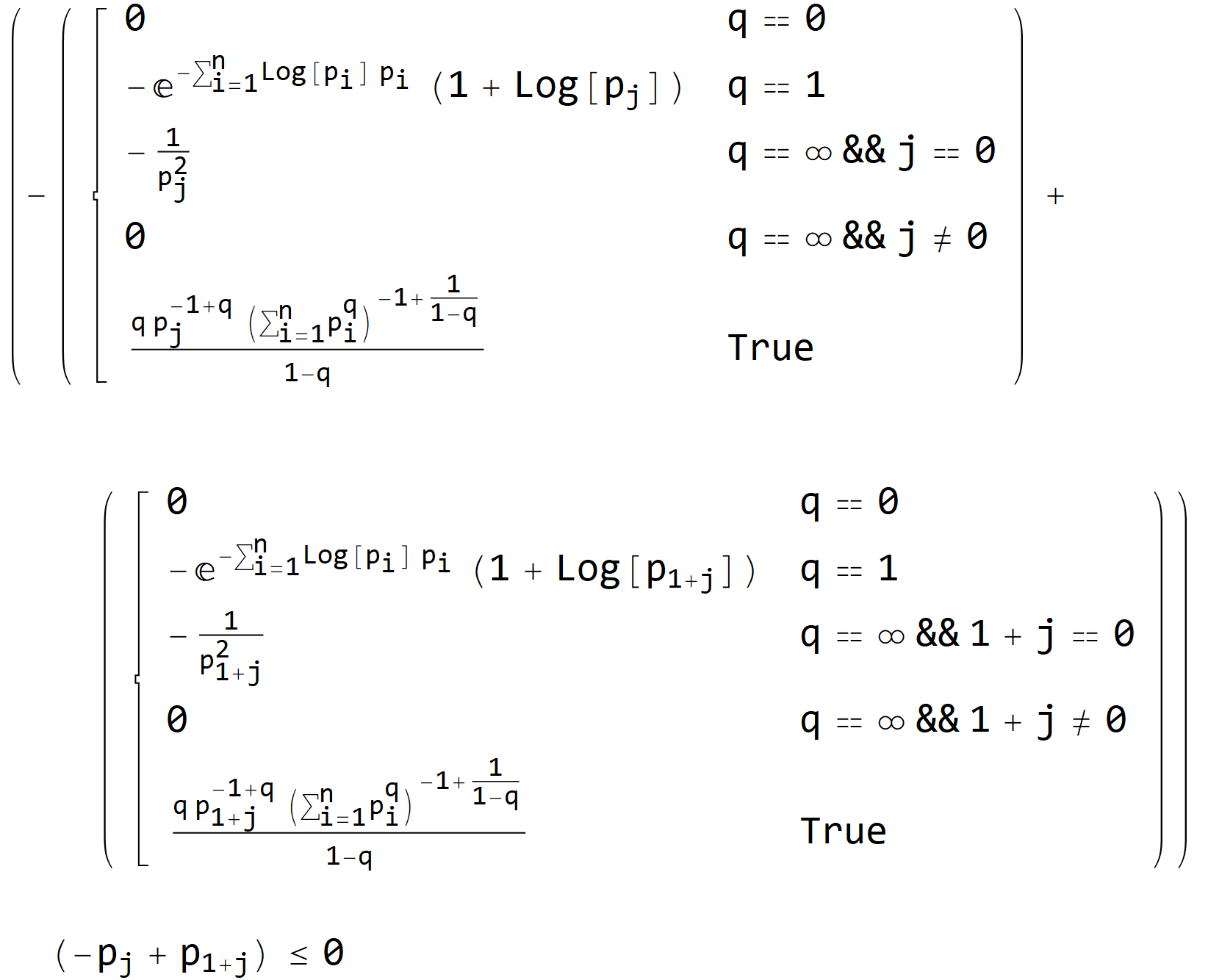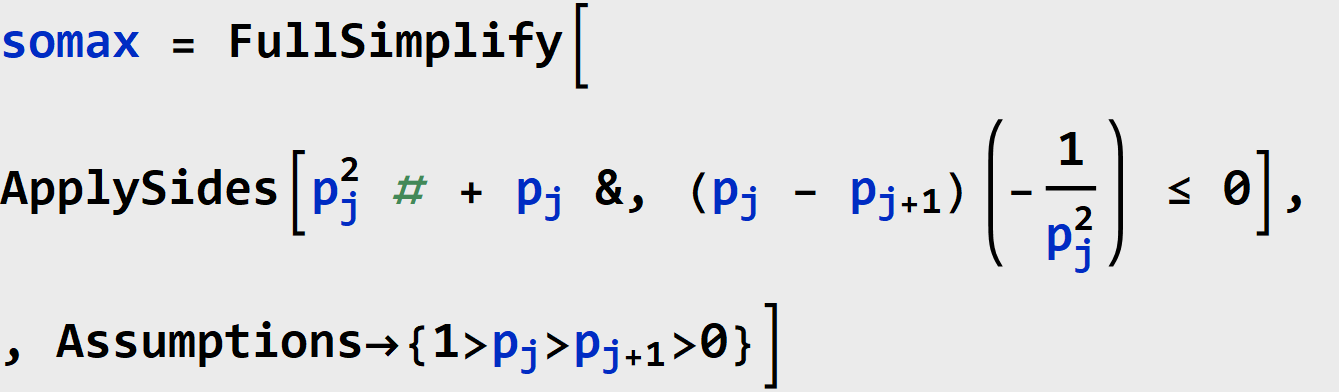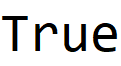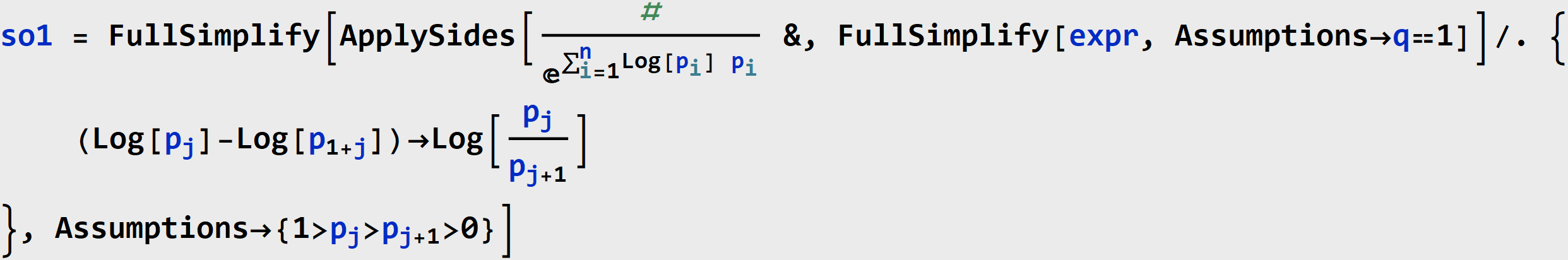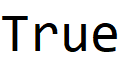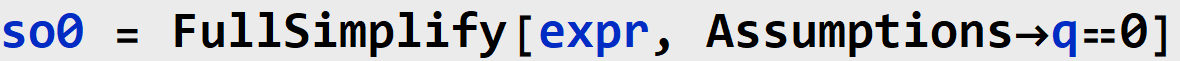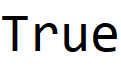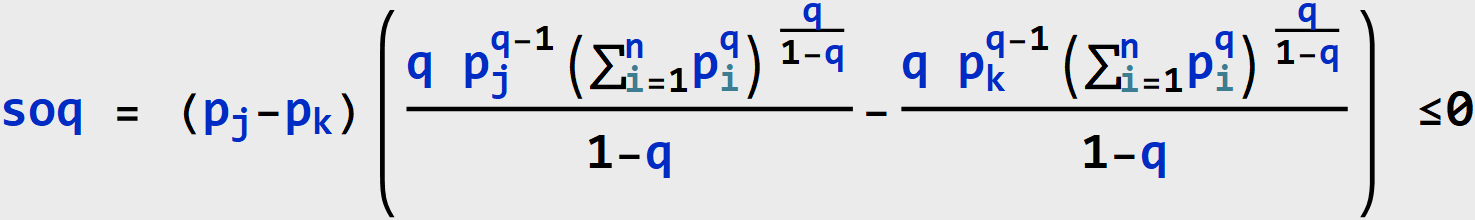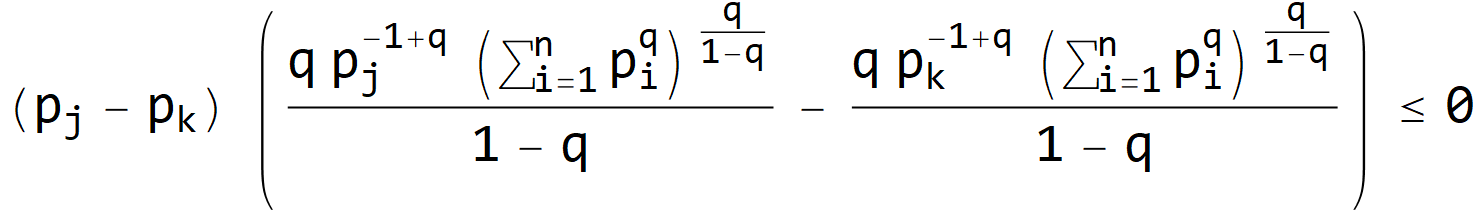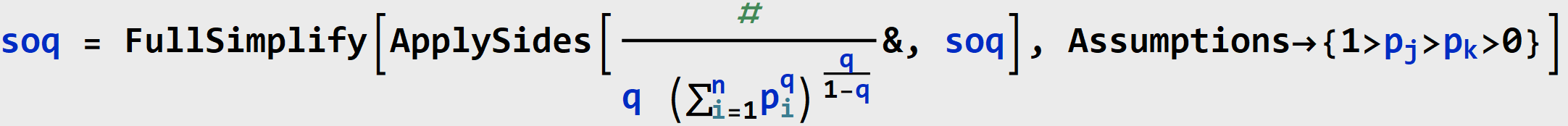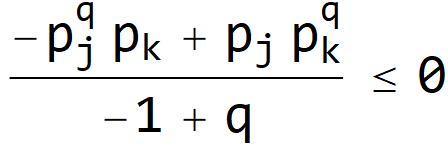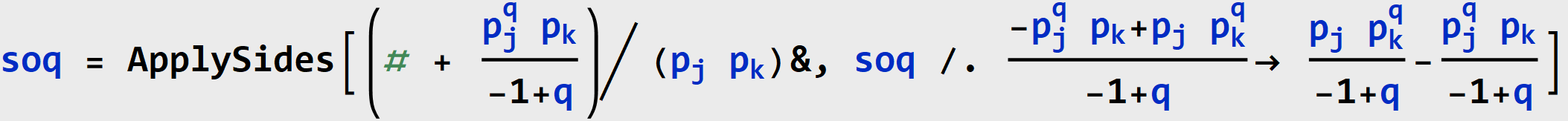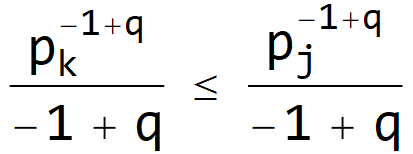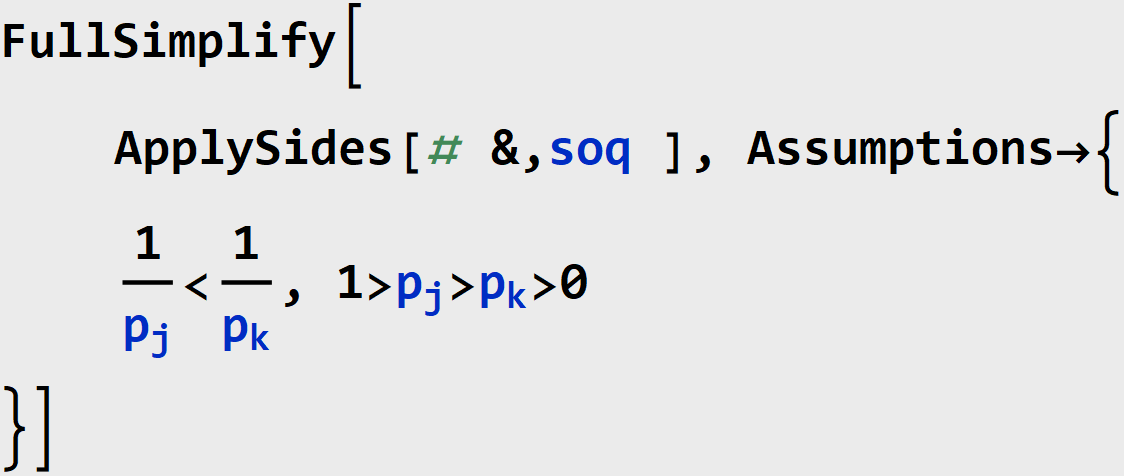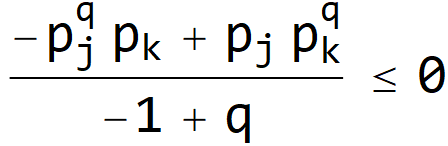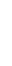Schur-Concavity of Rényi Heterogeneity

Abraham Nunes MD PhD MBA
Dalhousie University, Halifax, Nova Scotia, Canada

The Rényi heterogeneity of system X defined on state space X={1,2,..., n}, with probability distribution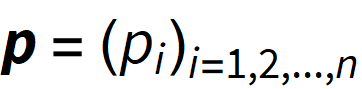is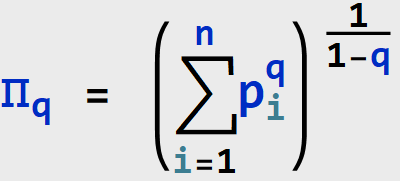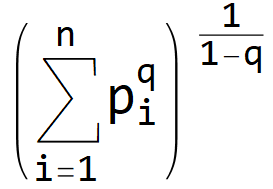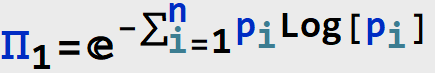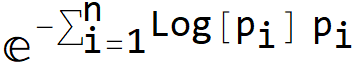The Schur-Ostrowski criterion states that if f is a symmetric function and all first partial derivatives exist, then f is Schur-concave iff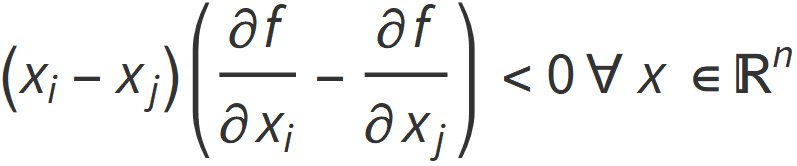holds for all 1ij<n

PROPOSITION 1 (Symmetry of Rényi Heterogeneity). Given a probability vector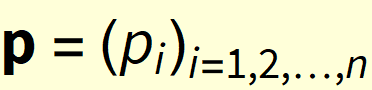and permutation function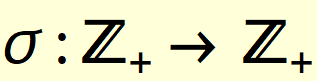, the Rényi heterogeneity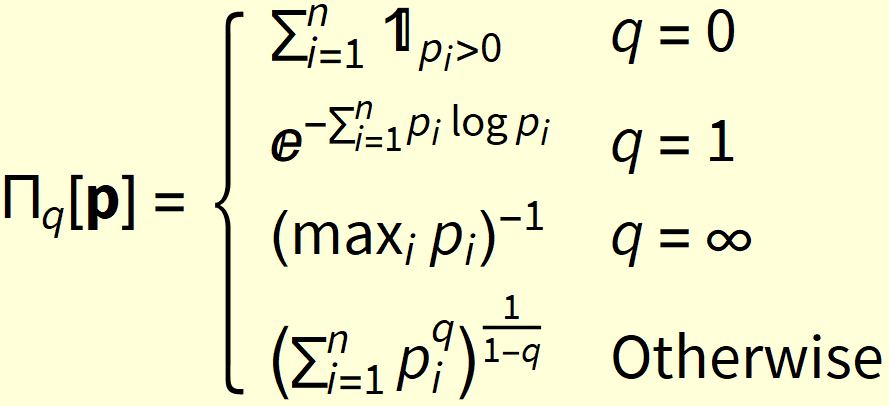,

satisfies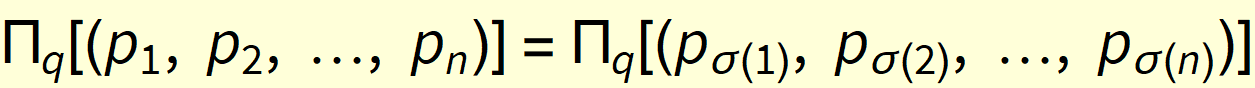.

The proof trivially follows from the commutativity of addition.

PROPOSITION 2 (Differentiability). Given a probability vector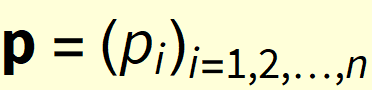and the Rényi heterogeneity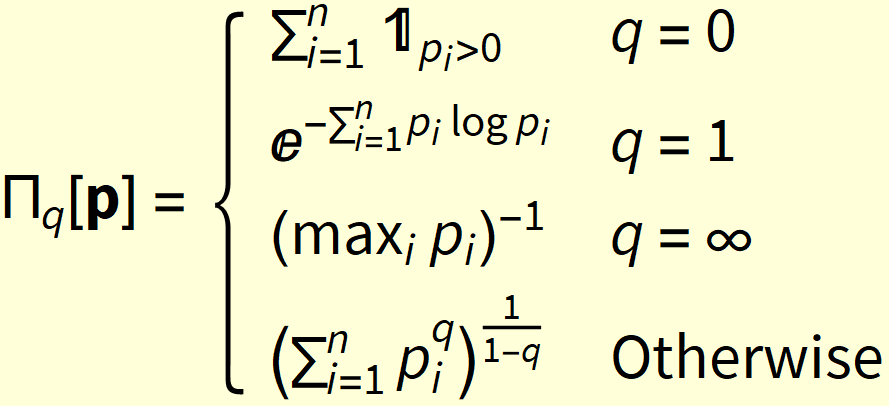,

we have that ∃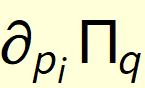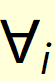.

Proof.  At q∉{0,1,∞}, we have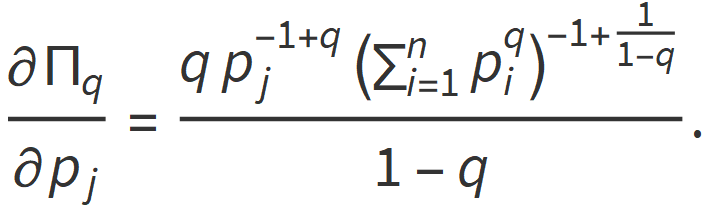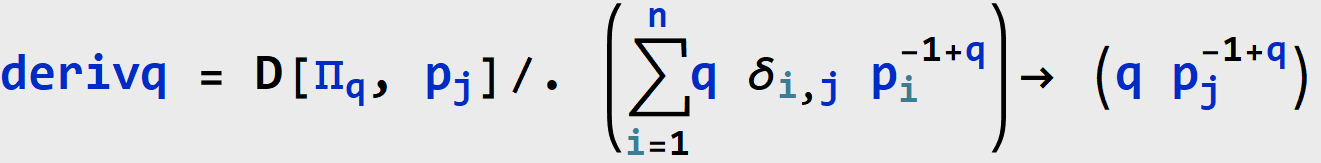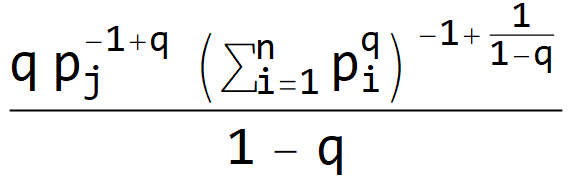At q=0, we have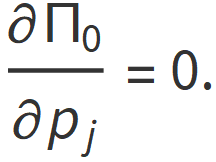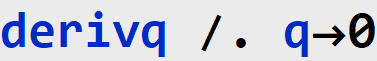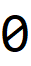At q=1 we have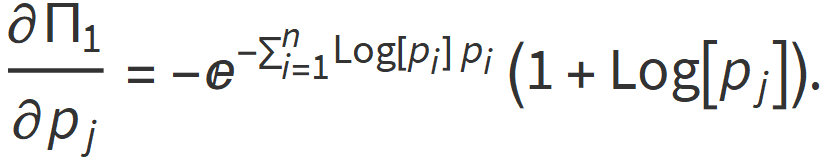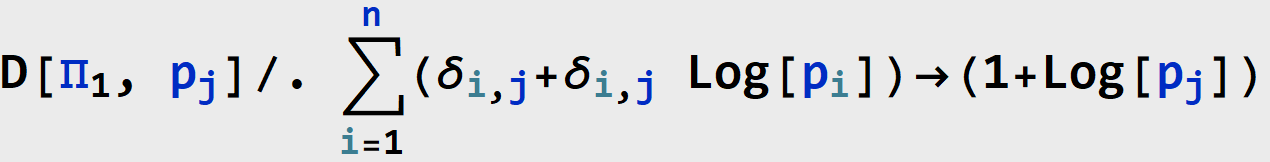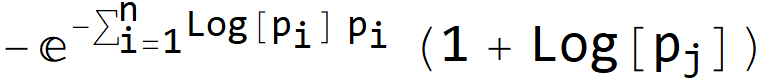At q= we have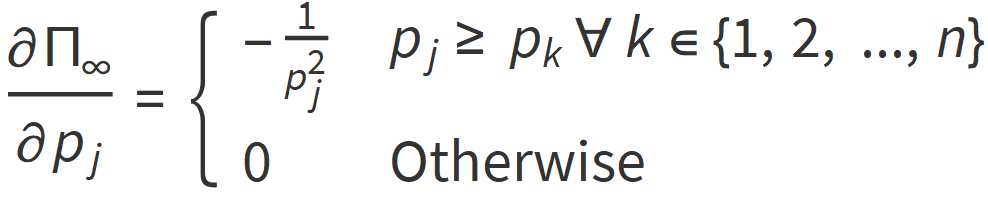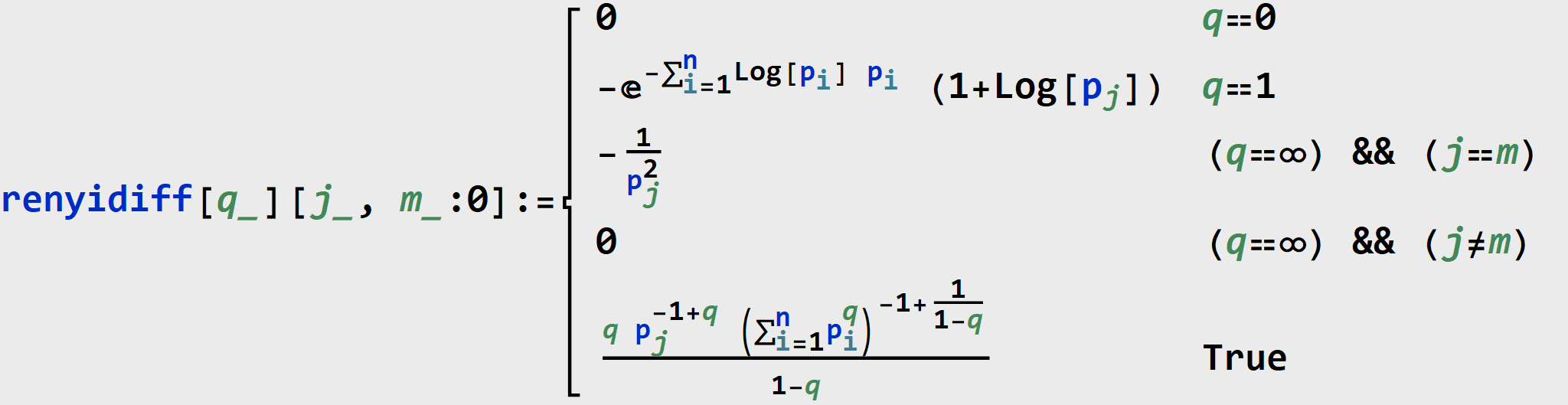THEOREM (Schur-Concavity of Rényi Heterogeneity). The Rényi heterogeneity is Schur-concave.

Proof.